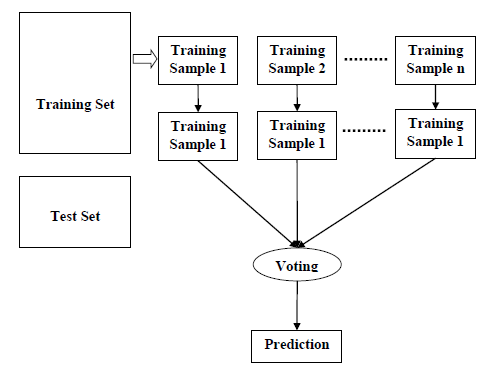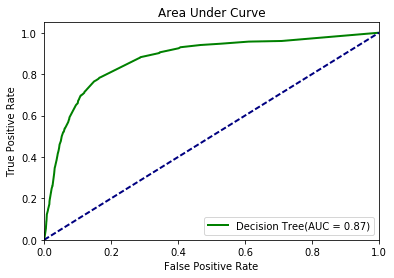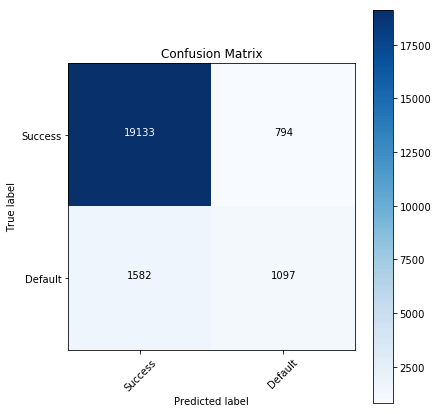# Random Forest Model : RFM

By Learnbay Category Machine Learning Reading time 9-12 mins Published on Dec 20, 2020

## Random Forest Model: Introduction

Random Forest Model is also a classification model with the combination of the decision tree. The random forest model algorithm is a supervised classification algorithm. As the name suggests, this algorithm creates the forest with several trees. … In the same way in the random tree classifier, the higher the number of trees in the forest gives the high the accuracy results. If you know the Random forest algorithm is a supervised classification algorithm.

The random forest model follows an ensemble technique. It involves constructing multi decision trees at training time. Its prediction is based on mode for classification and means for regression tree. It helps to reduce the overfitting of the individual decision tree. There are many possibilities for the occurrence of overfitting.

## Random Forest Model Algorithm: Working

We can understand the working of the Random Forest algorithm with the help of following steps −

• Step 1 − First, start with the selection of random samples from a given dataset. Do sampling without replacement.Sampling without replacement stats that the training data split into several small samples and then the result we get is a combination of all the data set. If we have 1000 features in a data set the splitting will happen with 10 features each in a small training data and all split training data contains equal no of features. The result is based on which training data has the highest value.

• Step 2 − Next, this algorithm will construct a decision tree for every sample. Then it will get the prediction result from every decision tree.
• Step 3 − In this step, voting will be performed for every predicted result.
• Based on ‘n’ samples… ‘n’ tree is built
• Each record is classified based on the n tree
• The final class for each record is decided based on voting

Step 4 − At last, select the most voted prediction result as the final prediction result.

## What is the Out of Bag score in Random Forests?

Out of bag (OOB) score is a way of validating the Random forest model. Below is a simple intuition of how is it calculated followed by a description of how it is different from the validation score and where it is advantageous.

For the description of the OOB score calculation, let’s assume there are five DTs in the random forest ensemble labeled from 1 to 5. For simplicity, suppose we have a simple original training data set as below.

OOB Error Rate Computation Steps

• Sample left out (out-of-bag) in Kth tree is classified using the Kth tree
• Assume j cases are misclassified
• The proportion of time that j is not equal to true class averaged over all cases is the OOB error rate.

Variable importance of RF:

It stats about the feature that is most useful for the random forest model by which we can get the high accuracy of the model with less error.

• Random Forest computes two measures of Variable Importance
• Mean Decrease in Accuracy
• Mean Decrease in Gini
• Mean Decrease in Accuracy is based on permutation
• Randomly permute values of a variable for which importance is to be computed in the OOB sample
• Compute the Error Rate with permuted values
• Compute decrease in OOB Error rate (Permuted- Not permuted)
• Average the decrease overall the trees
• Mean Decrease in Gini is computed as a “total decrease in node impurities from splitting on the variable averaged over all trees”.

Finding the optimal values using grid-search cv:

It stats the optimal values of the splitting decision tree that how many trees to be split within the model.

Measuring RF model performance by Confusion Matrix:

A confusion matrix is a table that is often used to describe the performance of a classification model (or “classifier”) on a set of test data for which the true values are known. It allows the visualization of the performance of an algorithm. It tells about how many true values are true.

Random Forest with python:

Importing the important libraries–

import pandas as pd

import numpy as np

from sklearn import preprocessing

from sklearn.model_selection import train_test_split

from sklearn.tree import DecisionTreeClassifier

from sklearn.metrics import confusion_matrix

from sklearn.metrics import accuracy_score

from sklearn import svm

from sklearn.metrics import roc_curve, auc

import matplotlib.pyplot as plt

from sklearn.externals.six import StringIO

from IPython.display import Image

from sklearn.tree import export_graphviz

temp = dummy_df.columns.values temp

print(dummy_df)

## Data Pre-Processing:

columns_name = temp.split(';')

data = dummy_df.values

print(data)

print(data.shape)

contacts = list()

for element in data:

contact = element.split(';')

contacts.append(contact)

contact_df = pd.DataFrame(contacts,columns = columns_name)

print(contact_df)

def preprocessor(df):

res_df = df.copy()

le = preprocessing.LabelEncoder()

encoded_df = preprocessor(contact_df)

#encoded_df = preprocessor(contacts)

x = encoded_df.drop(['"y"'],axis =1).values

y = encoded_df['"y"'].values

## Split the data into Train-Test¶

x_train, x_test, y_train, y_test = train_test_split(x,y,test_size =0.5)

# Decision tree with depth = 2

model_dt_2 = DecisionTreeClassifier(random_state=1, max_depth=2)

model_dt_2.fit(x_train, y_train)

model_dt_2_score_train = model_dt_2.score(x_train, y_train)

print("Training score: ",model_dt_2_score_train)

model_dt_2_score_test = model_dt_2.score(x_test, y_test)

print("Testing score: ",model_dt_2_score_test)

#y_pred_dt = model_dt_2.predict_proba(x_test)[:, 1] #Decision tree

model_dt = DecisionTreeClassifier(max_depth = 8, criterion ="entropy")

model_dt.fit(x_train, y_train)

y_pred_dt = model_dt.predict_proba(x_test)[:, 1]

## Graphical Representation of Tree

plt.figure(figsize=(6,6))

dot_data = StringIO()

export_graphviz(model_dt, out_file=dot_data,

filled=True, rounded=True,

special_characters=True)

graph = pydotplus.graph_from_dot_data (dot_data.getvalue())

Image(graph.create_png())

## Performance Metrics

fpr_dt, tpr_dt, _ = roc_curve(y_test, y_pred_dt)

roc_auc_dt = auc(fpr_dt, tpr_dt)

predictions = model_dt.predict(x_test)

# Model Accuracy

print (model_dt.score(x_test, y_test))

y_actual_result = y_test for i in range(len(predictions)):

if(predictions[i] == 1):

y_actual_result = np.vstack((y_actual_result, y_test[i]))

## Recall

#Recall

y_actual_result = y_actual_result.flatten()

count = 0

for result in y_actual_result:

if(result == 1):

count=count+1

print ("true yes|predicted yes:")

print (count/float(len(y_actual_result)))

## Area Under the Curve

plt.figure(1)

lw = 2

plt.plot(fpr_dt, tpr_dt, color='green',

lw=lw, label='Decision Tree(AUC = %0.2f)' % roc_auc_dt)

plt.plot([0, 1], [0, 1], color='navy', lw=lw, linestyle='--')

plt.xlim([0.0, 1.0])

plt.ylim([0.0, 1.05])

plt.xlabel('False Positive Rate')

plt.ylabel('True Positive Rate')

plt.title('Area Under Curve')

plt.legend(loc="lower right")

plt.show()## Confusion Matrix

print (confusion_matrix(y_test, predictions))

accuracy_score(y_test, predictions)

import itertools

from sklearn.metrics import confusion_matrix

def plot_confusion_matrix(model, normalize=False): # This function prints and plots the confusion matrix.

cm = confusion_matrix(y_test, model, labels=[0, 1])

classes=["Success", "Default"] cmap = plt.cm.Blues

title = "Confusion Matrix"

if normalize:

cm = cm.astype('float') / cm.sum(axis=1)[:, np.newaxis] cm = np.around(cm, decimals=3)

plt.imshow(cm, interpolation='nearest', cmap=cmap)

plt.title(title)

plt.colorbar()

tick_marks = np.arange(len(classes))

plt.xticks(tick_marks, classes, rotation=45)

plt.yticks(tick_marks, classes)

thresh = cm.max() / 2.

for i, j in itertools.product(range(cm.shape), range(cm.shape)):

plt.text(j, i, cm[i, j],

horizontalalignment="center",

color="white" if cm[i, j] > thresh else "black")

plt.tight_layout()

plt.ylabel('True label')

plt.xlabel('Predicted label')

plt.figure(figsize=(6,6))

plot_confusion_matrix(predictions, normalize=False)

plt.show()### Pruning of the tree¶

from sklearn.tree._tree import TREE_LEAF

def prune_index(inner_tree, index, threshold):

if inner_tree.value[index].min() < threshold:

# turn node into a leaf by "unlinking" its children

inner_tree.children_left[index] = TREE_LEAF

inner_tree.children_right[index] = TREE_LEAF

# if there are shildren, visit them as well

if inner_tree.children_left[index] != TREE_LEAF:

prune_index(inner_tree, inner_tree.children_left[index], threshold)

prune_index(inner_tree, inner_tree.children_right[index], threshold)

print(sum(model_dt.tree_.children_left < 0))

# start pruning from the root

prune_index(model_dt.tree_, 0, 5)

sum(model_dt.tree_.children_left < 0)

#It means that the code has created 17 new leaf nodes

#(by practically removing links to their ancestors). The tree, which has looked before like

from sklearn.externals.six import StringIO

from IPython.display import Image

from sklearn.tree import export_graphviz

import pydotplus

plt.figure(figsize=(6,6))

dot_data = StringIO()

export_graphviz(model_dt, out_file=dot_data,

filled=True, rounded=True,

special_characters=True)

graph = pydotplus.graph_from_dot_data (dot_data.getvalue())

Image(graph.create_png())

Learnbay provides industry accredited data science courses in Bangalore. We understand the conjugation of technology in the field of Data science hence we offer significant courses like Machine Learning, Tensor Flow, IBM Watson, Google Cloud platform, Tableau, Hadoop, time series, R and Python. With authentic real-time industry projects. Students will be efficient by being certified by IBM. Around hundreds of students are placed in promising companies for data science roles. Choosing Learnbay you will reach the most aspiring job of present and future.

Learnbay data science course covers Data Science with Python, Artificial Intelligence with Python, Deep Learning using Tensor-Flow. These topics are covered and co-developed with IBM.